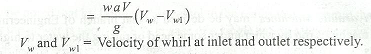## Featured Post

### Best Schools for Mechanical Engineering Degree | University Ranking

Interested in studying from top mechanical engineering schools and institutes in the world? We have compiled a list of best mechanical engin...### Impact of Water Jets

What is the impact of jets of water in hydraulic machines and fluid dynamics? What force does a water jet imposes on different surfaces like plane surface, curved surface and moving curved vanes etc.

The following relations are important :

(a) Force exerted by a jet of water impinging normally on a fixed plate,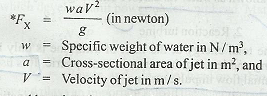(b) Force exerted by a jet of water impinging normally (i.e. perpendicular) on a fixed plate inclined at an angle 0 (as shown in Fig. 4.1) is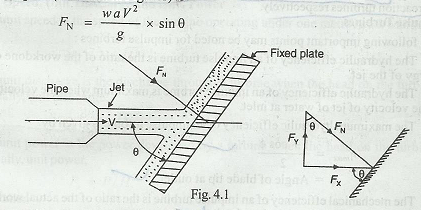Force exerted by the jet in the direction of flow,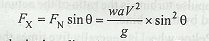and force exerted by the jet in a direction normal to flow,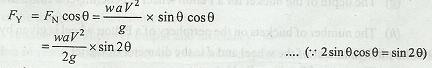(c) When a jet of water enters and leaves the curved fixed plate or vane tangentially, then the force of the jet along normal to the plate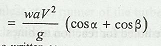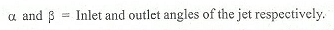(d) Force exerted by a jet of water impinging normally on a plate which due to the impact of jet, moves in the direction of jet with a velocity v is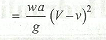(e) When a jet of water enters and leaves a moving curved vane, then the force of jet in the direction of motion of the vane is